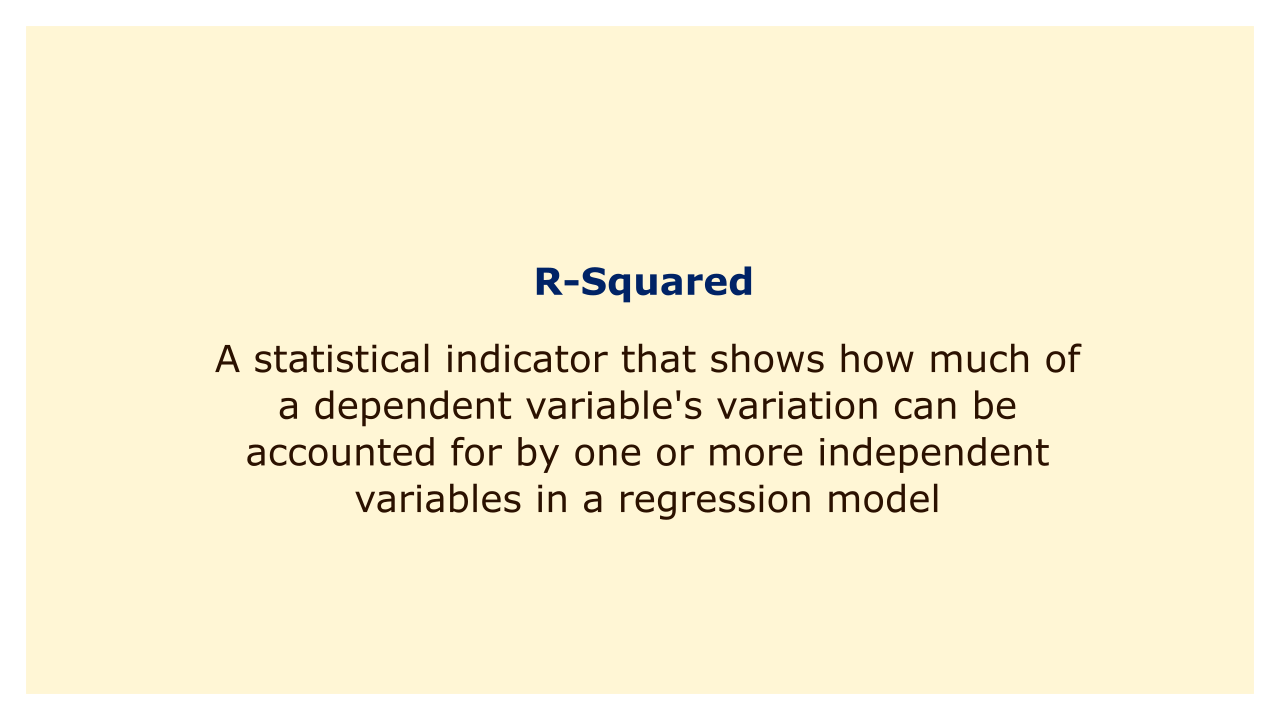# R-SquaredImage: Moneybestpal.com

### R-squared, commonly referred to as the coefficient of determination, is a statistical indicator that shows how much of a dependent variable's variation can be accounted for by one or more independent variables in a regression model.

The R-squared value ranges from 0 to 1, with a value of 1 denoting that all variance in the dependent variable is explained by the model's independent variables and a value of 0 denoting that none of the variations in the dependent variable is.

R-squared is a crucial statistic in regression analysis since it aids in assessing the regression model's goodness of fit. A high R-squared value denotes a good fit between the model and the data, whereas a low R-squared value denotes a poor match between the model and the data. It is crucial to remember that just because a model has a high R-squared value, it does not necessarily mean that it is a strong predictor of future results. This is because there may be additional elements that the model does not take into account that could have an impact on future outcomes.

Remember that the number of independent variables in a model can have an impact on R-squared, and that including more independent variables in a model might enhance its R-squared value even if those additional factors have a negligible effect on the dependent variable. In order to properly assess the effectiveness of regression models with numerous independent variables, it is crucial to take into account other metrics, such as adjusted R-squared.
Tags